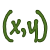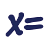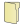Games
Problems
Go Pro!

# Equations

Pro Problems > Math > Algebra > Equations

# Featured Pro ProblemsFind the sum of x and y, if the following quadratic equations in x and y are true.Two people go to the same store and make the same purchases, but one of them is treated unfairly in the pricingRational expressions word problemThe sum of two numbers is six more than twice the smaller number, and if the larger number is multiplied by three, the result is ten less than seven...A hypothetical problem in which the treasury introduces a new coin into circulationDetermine how many floors a skyscraper has, based on information about someone climbing the stairsGiven three secret numbers, and information about each of them, find the numbersA messy one-variable word problem about how old James is# Full Directory Listing

Parenthetical Mess, Solve the CubicLike us on Facebook to get updates about new resources